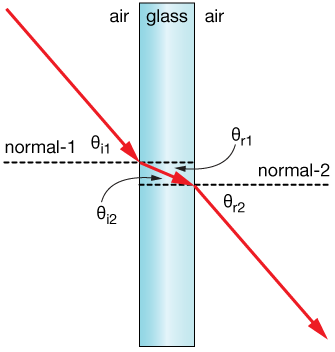# Total Internal Reflection in Optical Fibre

• Astrophysics• Electricity• Electromagnetism• Energy• Fields• Force• Mechanics• Momentum• Nuclear Physics• Quantities & Units• States of Matter• Waves• Key ExperimentsHave you ever watched a video of an endoscopy and wondered how doctors are able to see inside the human body? The answer in something called total internal reflection, which is also used in fiber optics. This principle allows doctors to see inside the body using an endoscope. But, total internal reflection is not just used in medicine. It's also used in other everyday applications.

## What is total internal reflection?

Total internal reflection occurs when light in a medium reflects completely back into the medium from its surroundings. The critical angle θc is the incident angle that produces an angle of refraction of 90°. The refractive index n determines the speed of light in the medium and is inversely proportional to it. In the diagram below, we see a light beam traveling from a medium with a refractive index of n1 to a second medium with a refractive index of n2, where n2 < n1. This is related to Total Internal Reflection in Optical Fibre.The behaviour of a light beam related to the refractive index and the angle of incidence

When we use mirrors in our daily lives, they reflect about 90% of the light and absorb the remaining portion, which is refracted as shown in Figure 1 (a). The refraction angle θ2 is greater than the incident angle θ1 because the refractive index of the second boundary n2 is less than the refractive index of the first boundary. As the incident angle θ1 increases, so does the refraction angle θ2, but it can only go up to 90° when the incident angle equals the critical angle θc (Figure 1 (b)). According to Snell's law, the ratio of the sines of the incident and refraction angles is constant between two mediums. When the incident angle equals the critical angle, or θ1 = θc, the refraction angle is 90° and sin90° = 1. Therefore, the critical angle between the boundaries of two materials where n1 > n2 can be determined using the following equation:

We should note that two conditions need to be fulfilled for total internal reflection of light to occur: (1) the incident angle must be greater than the critical angle (θ1 > θc), and (2) the refractive index of the initial medium must be greater than the refractive index of the second medium (n1 > n2). This is related to Total Internal Reflection in Optical Fibre.

## What are optical fibres?

Optical fibres or fibre optics have applications in a variety of fields, such as communications to transmit telephone signals, cable signals, TV signals, the internet, and medical signals. These fibres are very thin, which allows light to enter from one side and strike the inside of the fibre with an angle greater than the critical angle, which causes them to travel through the fibre and exit it at the same angle as the entry angle.

Cladding is the coating outside of optical fibres that have a refractive index that is smaller than the material inside the optical fibre and thus prevents light from being refracted between the fibres in a bundle.

While light with an incidence angle greater than the maximum acceptance angle θa is partially refracted outside the fibre, light with an incidence angle greater than the maximum acceptance angle θa is partially refracted inside the fibre. After several encounters with the fibre core-cladding interface, the residual, partially reflected light is finally lost. The requirement for 100% internal reflection at the core-cladding contact determines the maximum acceptance angle θa.To determine the acceptance angle θa, we should look at the equation that yields the critical angle θc. As you can see in Figure 2, θc' = 90°-θc. The equation thus helps us to determine the acceptance angle θa, assuming the fibre is in the air with a refractive index of 1. This can be expressed as follows: As noted before, the light exits the fibre at the same angle at which it entered it, which means that θa = θa'.

Total Internal Reflection In Optical Fiber - Key takeaways Total internal reflection is a phenomenon of light that occurs when the angle between the incident light and the normal of the boundary θ1 is greater than the critical angle θc.An optical fibre is a nonconducting waveguide that transmits light along its axis by total internal reflection. Optical fibres or fibre optics have applications in a variety of fields, such as communications to transmit telephone signals, cable TV signals, the internet, and medical signals. For the total internal reflection of light to occur, the incident angle θ1 must be greater than the critical angle θc, and the initial medium’s refractive index n1 must be greater than the second medium’s index n2.

## Total Internal Reflection in Optical Fibre

What is total internal reflection in fibre optics?

Total internal reflection is a phenomenon of light that occurs when the angle between the incident light and the normal of the boundary θ1 is greater than the critical angle θc.

Why is there total internal reflection in an optical fibre?

Total internal reflection occurs in optical fibre because the refractive index of the coating material called cladding is less than the refractive index of the material inside the optical fibre.

What is the principle of total internal reflection?

Total internal reflection is a phenomenon of light that occurs when the angle between the incident light and the normal of the boundary θ1 is greater than the critical angle θc. In that case, no light is refracted into the second medium but all light is reflected back.14-day free trial. Cancel anytime.Join 10,000+ learners worldwide.The first 14 days are on us96% of learners report x2 faster learningFree hands-on onboarding & supportCancel Anytime